Function Repository Resource:

# AccumulateApply

Apply a function to progressively longer sequences from a list

Contributed by: Ian Ford and Jon McLoone
 ResourceFunction["AccumulateApply"][f,list] applies f to progressively longer sequences from list.

## Details

The list need not have a Head of List.

## Examples

### Basic Examples (2)

Apply an arbitrary function to progressive ranges of numbers:

 In:=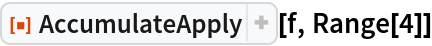Out=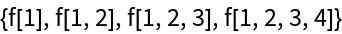Find all substrings from the start of a message:

 In:=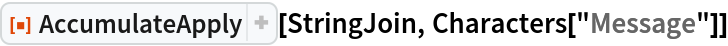Out=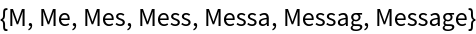### Scope (1)

The Head of the second argument need not be List:

 In:=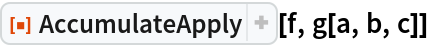Out=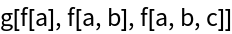### Applications (2)

Generate a sequence of factorials:

 In:=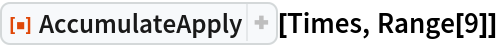Out=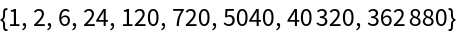In:=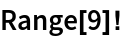Out=Find the running maximum of a list:

 In:=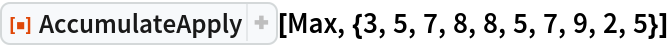Out=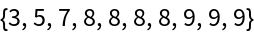### Properties and Relations (1)

AccumulateApply[Plus,list] is equivalent to Accumulate[list]:

 In:=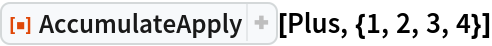Out=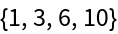In:=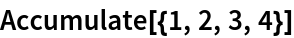Out=### Possible Issues (1)

Functions that expect a list should be composed with List:

 In:=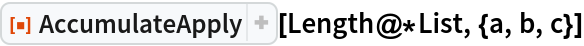Out=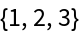Jon McLoone

## Version History

• 1.0.1 – 16 November 2021